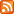RSS 1.0 XML Feed available

# Tweaking Analog Literals (C++ humor)

• Home
• Tweaking Analog Literals (C++ humor)

Date: 29-Aug-2009/18:37

Tags:

Characters: (none)

Jeremy Friesner brought this site about analog literals to my attention. It provides the long-needed ability to represent integer constants in C++ not as numbers (like 42) but rather as 1-D, 2-D, or 3-D shapes whose length, area, or volume correspond to the number's quantity. So for instance:
``````assert( ( o-------------o
|L             \
| L             \
|  L             \
|   o-------------o
|   !             !
!   !             !
o   |             !
L  |             !
L |             !
L|             !
o-------------o ).volume ==

( o-------------o
|             !
!             !
!             !
o-------------o ).area * int(I-------------I) );
``````
That's great! As the inventor of Arecibo ASCII, I fully support this visual double-check with our intuitions about numbers! What if aliens are trying to read our code, but don't know about our arbitrary choices of digits and numeric base?? This could bridge that important gap! :P
But there's one nagging concern I have, which is that I don't think the 1-D numeric values are very intuitive. Look at these examples from the site:
``````assert( I-I == 0 );
assert( I---I == 1 );
assert( I-----I == 2 );
assert( I-------I == 3 );
``````
I'd prefer it to more consistently depict the historic concept of zero, and be less arbitrary with the "2N+1" formula of dashes to implement value N. So why not overload dereference and multiply, and define "II" to be the constant value zero? This way you can get:
``````assert(II == 0);
assert(I*I == 1);
assert(I**I == 2);
assert(I***I == 3);
``````
The implementation is relatively straightforward from the proposal. But I went ahead and wrote it, and it is complete enough to give errors when compiling invalid literal specifications:
``````int test1 (I); // compile error!
int test2 (*I); // compile error!
int test3 (I*); // compile error!
int test4 (*I*I); // compile error!
int test5 (I*I*); // compile error!
``````
I hope this makes it more practical for people to apply analog literals to real-world situations! Source below...
``````#include <cassert>

// Implementation for Tweaked 1-D Literal proposal

class Accumulator {
private:
int value;
public:
Accumulator(int newValue) : value (newValue)
{ }
Accumulator operator*() const
{ return Accumulator (value + 1); }
int getValue() const
{ return value; }
};

class Delimiter {
public:
Delimiter()
{ }
int operator*(const Accumulator& rhs) const
{ return rhs.getValue() + 1; }
int operator*(const Delimiter& rhs) const
{ return 1; }
Accumulator operator*() const
{ return Accumulator (1); }
};

const int II (0);
const Delimiter I;

// Tests for Tweaked 1-D Literal proposal

int main() {
assert(II == 0);
assert(I*I == 1);
assert(I**I == 2);
assert(I***I == 3);

#if 0
// these cause compilation errors, by design
int test1 (I);
int test2 (*I);
int test3 (I*);
int test4 (*I*I);
int test5 (I*I*);
#endif

return 0;
}
``````